# 学习笔记（2.17）

## 1.KNN水果分类实践

import pandas as pd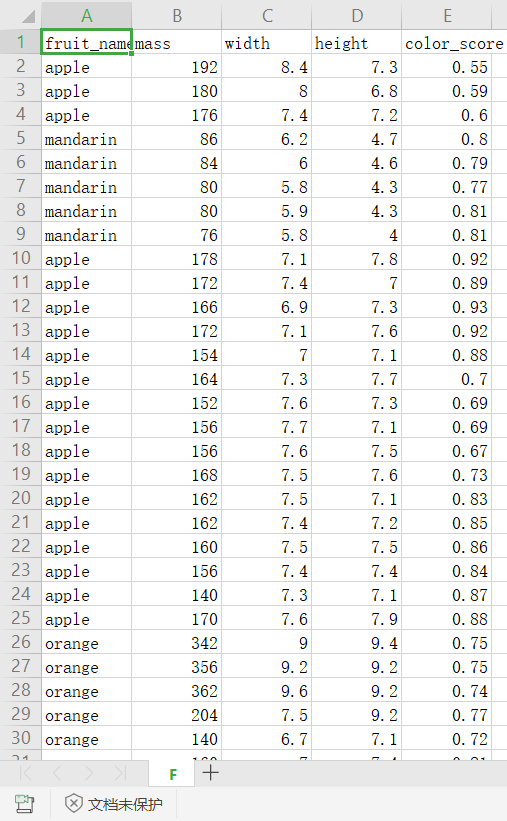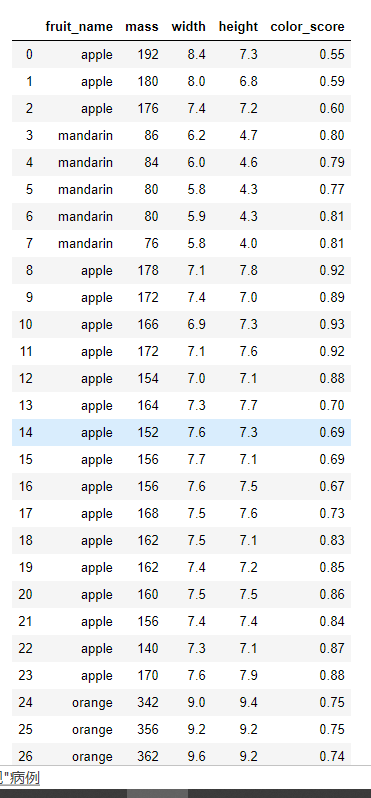2.labelencoder=LabelEncoder()
data.iloc[:,0]=labelencoder.fit_transform(data.iloc[:,0])

A.data.iloc 是从DataFrame中筛选数据可以根据行和列来进行筛选
B.使用 loc 从DataFrame中筛选数据是根据列头的标签来选择，在坐标轴上挑选不同的列头以获取希望的数据
C.使用 ix 进行选择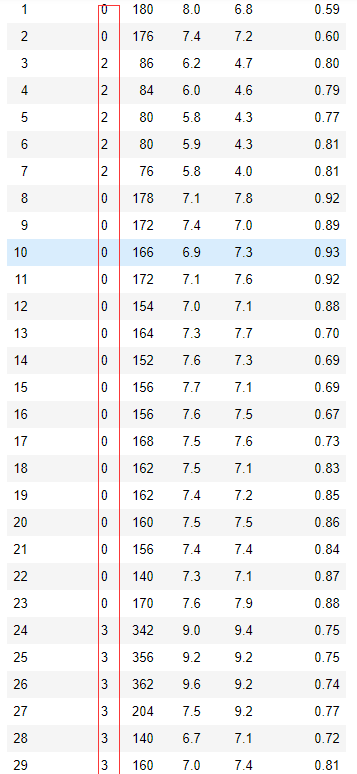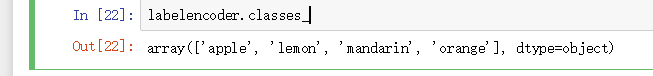3.data.iloc[:,1:]这个语法再一次困扰着我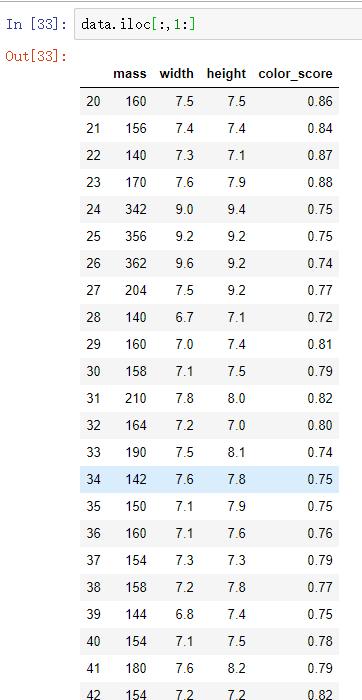4. knn=KNeighborsClassifier(i)
KNeighborsClassifier又称K最近邻，是一种经典的模式识别分类方法。sklearn库中的该分类器有以下参数：

from sklearn.neighbors import KNeighborsClassifier;

model = KNeighborsClassifier(
n_neighbors=5,
weights=’uniform’,
algorithm=’auto’,
leaf_size=30,
p=2,
metric=’minkowski’,
metric_params=None,
n_jobs=None,
**kwargs=object);



5.x_train,x_test,y_train,y_test=train_test_split(data.iloc[:,1:],data.iloc[:,0],test_size=0.3,stratify=data.iloc[:,0],random_state=20

6.整个过程其实不是很复杂，首先获取数据，把数据的标签和特征分开，把数据集中的数据切分为训练集和测试集，用循环的方式找到一个最优的K值放入KNN模型中得到最好分类精确度。

## BP神经网络手写数字识别

1.from sklearn.cross_validation import train_test_split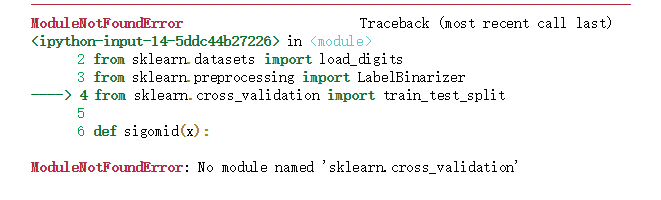from sklearn.model_selection import train_test_split


2.输入数据归一化

#输入数据归一化
X-=x.min()
X/=X.max()


3.标签二值化

0>1000000000
1>0100000000
2>0010000000
3>0001000000
4>0000100000

## BP 神经网络到底怎么回事还是没有搞清楚，继续战斗

posted on 2020-02-17 19:26  那我先走了  阅读(180)  评论(0编辑  收藏  举报